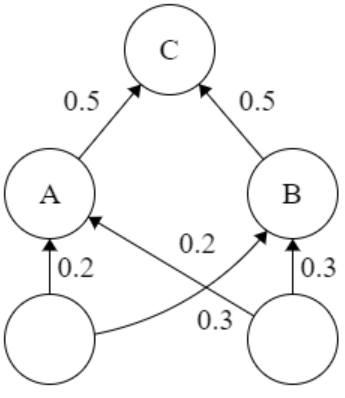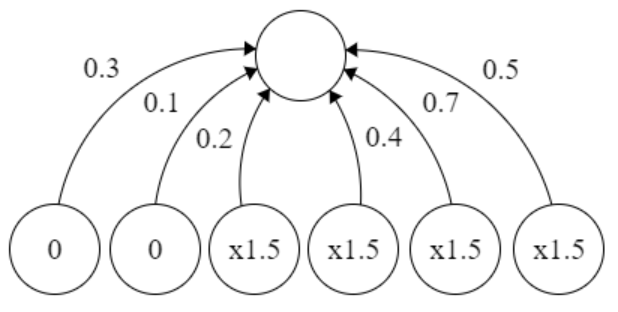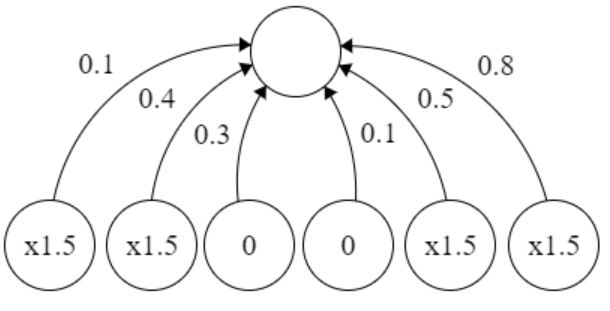# Dropout in Neural Networks

• Difficulty Level : Hard
• Last Updated : 20 Jun, 2022

The concept of Neural Networks is inspired by the neurons in the human brain and scientists wanted a machine to replicate the same process. This craved a path to one of the most important topics in Artificial Intelligence. A Neural Network (NN) is based on a collection of connected units or nodes called artificial neurons, which loosely model the neurons in a biological brain. Since such a network is created artificially in machines, we refer to that as Artificial Neural Networks (ANN). This article assumes that you have a decent knowledge of ANN. More about ANN can be found here. Now, let us go narrower into the details of Dropout in ANN.

## Python3

 tf.keras.layers.Dropout(    rate) # rate: Float between 0 and 1.# The fraction of the input units to drop.

Problem: When a fully-connected layer has a large number of neurons, co-adaption is more likely to happen. Co-adaptation refers to when multiple neurons in a layer extract the same, or very similar, hidden features from the input data. This can happen when the connection weights for two different neurons are nearly identical.This poses two different problems to our model:

• Wastage of machine’s resources when computing the same output.
• If many neurons are extracting the same features, it adds more significance to those features for our model. This leads to overfitting if the duplicate extracted features are specific to only the training set.

Solution to the problem: As the title suggests, we use dropout while training the NN to minimize co-adaption. In dropout, we randomly shut down some fraction of a layer’s neurons at each training step by zeroing out the neuron values. The fraction of neurons to be zeroed out is known as the dropout rate,. The remaining neurons have their values multiplied byso that the overall sum of the neuron values remains the same.The two images represent dropout applied to a layer of 6 units, shown at multiple training steps. The dropout rate is 1/3, and the remaining 4 neurons at each training step have their value scaled by x1.5. Thereby, we are choosing a random sample of neurons rather than training the whole network at once. This ensures that the co-adaption is solved and they learn the hidden features better.

Why dropout works?

• By using dropout, in every iteration, you will work on a smaller neural network than the previous one and therefore, it approaches regularization.
• Dropout helps in shrinking the squared norm of the weights and this tends to a reduction in overfitting.

Dropout can be applied to a network using TensorFlow APIs as follows:

## Python3

 tf.keras.layers.Dropout(    rate) # rate: Float between 0 and 1.# The fraction of the input units to drop.

My Personal Notes arrow_drop_up